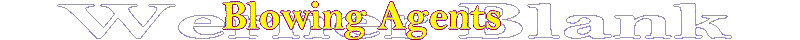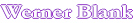Thermal Conductivity

Home

Thermal conductivity of a gas is of great importance for the insulating properties of a foam. In a foam the blowing agent (gas) accounts for approximately 50 % of the heat conductivity. The nature of the blowing agent is therefore important to achieve high insulating properties

kT=kg+ks+kr ;  heat conductivity, T=total, g=gas, s=solids, r=radiant

Thermal Conductivity - Thermal conductivity is a measure of the ability of a material to conduct heat . This ability is determined by the material's composition, i.e., the type and ratio of thermal filler to elastomeric binder, and by the relative amount of reinforcing metal foil, glass fiber or dielectric film. Thermal conductivity is usually expressed in units of Watt/m-K, where,

W/m-K = (Cal/sec-cm-°C x 420) = (BTU-in/hr-ft2-°F x 0.14)

As shown in this table for high insulating properties of a foam gases with a low thermal conductivity are required. CFC-11 was an ideal gas from a thermal conductivity viewpoint. Carbon dioxide although very desirable from an environmental standpoint as a blowing agent, is not desirable in insulating foams from an insulating viewpoint. Because carbon dioxide is used as a co-blowing agent in the preparation of refrigeration foams to achieve a higher strength and better flow of the foams have higher heat conductivity. Carbon dioxide can be remove by in-situe absorption with a strong base. In open systems containing fluorocarbon blowing agents  due to diffusion and exchange with air the thermal conductivity will decline and approach the value of nitrogen/oxygen.

GAS

Mw

Thermal Conductivity k BTU-in/hr-ft2-°F

Xenon

131*

0.039

Trichlorofluoromethane  CFC-11

137.4

0.053

Krypton

83.8*

0.056

1,1-Dichloro-1-fluoro-ethane HCFC 141b

117

0.074

70.1

0.083

Sulfur hexafluoride SF6

146

0.093

1,1,1,2-Tetrafluoroethane HFC-134a

102

0.101

Isopentane

72.2

0.102

72.2

0.105

Butane

58.1

0.111

Isobutane

58.1

0.112

44.0

0.115

Argon

39.9*

0.123

Nitrogen

28*

0.179

Oxygen

32*

0.184

Air is average between Nitrogen/Oxygen

* Atomic weight

Some conversion factors

Quantity

S.I. Units

=

Multiplier

x

Other Units

Heat flux

W/m2

=

3.154

x

BTU/hr-ft2

Heat transfer coefficient

W/m2-K

=

5.6786

x

BTU/hr-ft2-F

Specific heat

J/kg-K

=

4186.9

x

BTU/lbm-F

J/kg-K

=

4186.8

x

cal/g-C

Thermal conductivity

W/m-K

=

1.7307

x

BTU/hr-ft-F

W/m-K

=

418.68

x

cal/s-cm-C

Last edited on:

November 16, 2006

Copyright®, Design, Layout and Technical Content by: